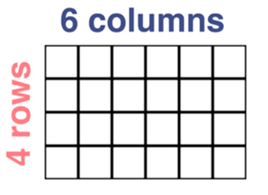Mathematics
Easy

Question

# 4 × 6=_______.## 10    24    16    20Hint:

## The correct answer is: 24

### In the question there is a table using which we have to find the multiplication expression.The number of columns in the given table= 6The number of rows in the given table= 4The multiplication expression of the given table= 4×6=24.So, the multiplication expression of the given table is 4×6=24.Therefore, the correct option is b, i.e., 24.

For simple multiplication we have to remember the multiplication table of one-digit numbers and for two-digit number we can find it using the multiplication table of one-digit numbers. Here, we have to find the product of one digit number.

### Related Questions to study#### With Turito Foundation.#### Get an Expert Advice From Turito.